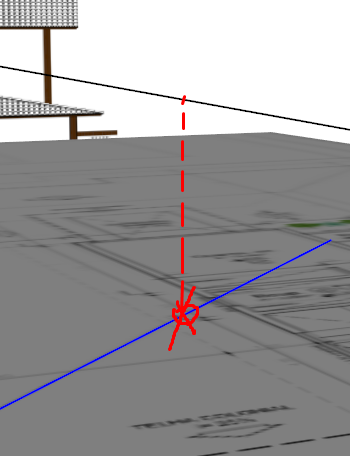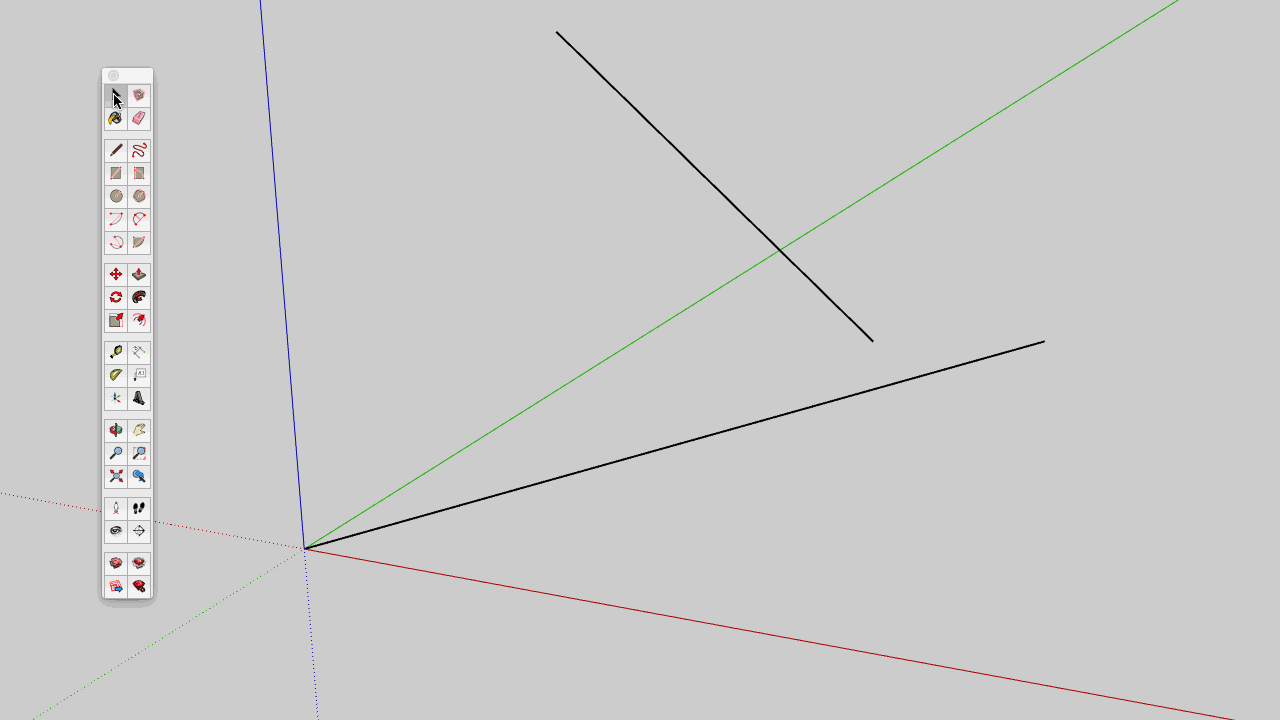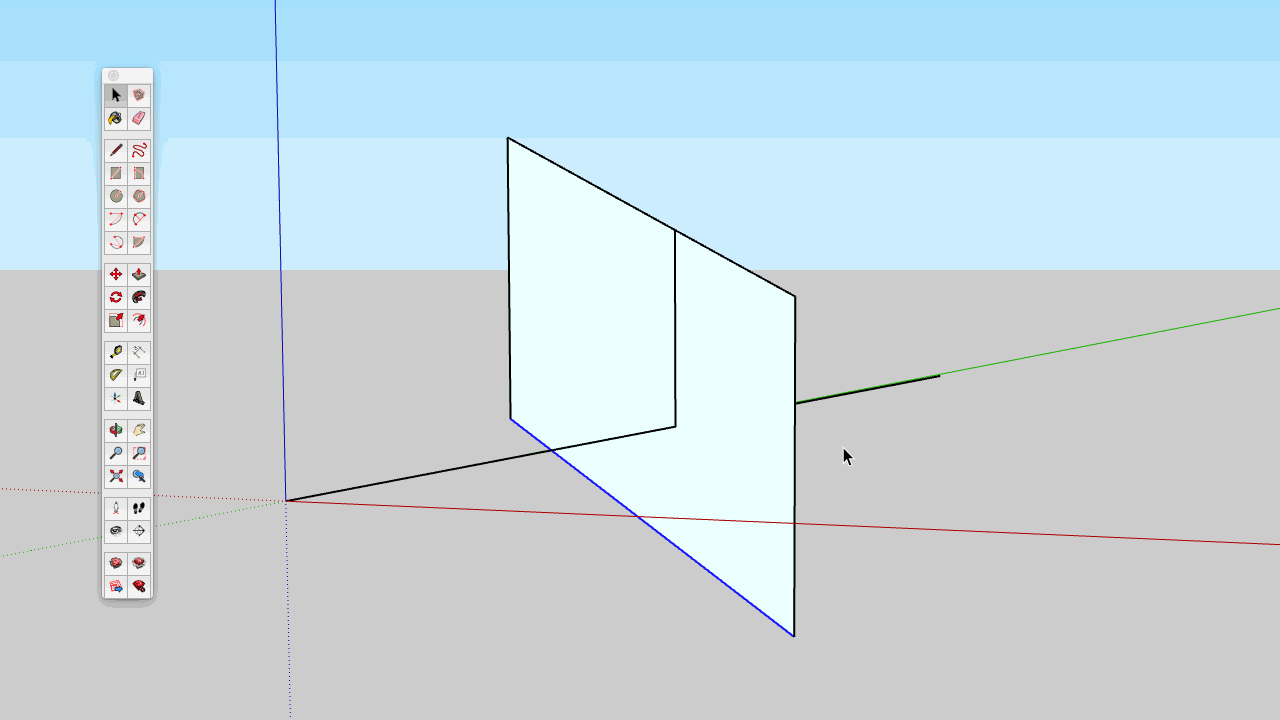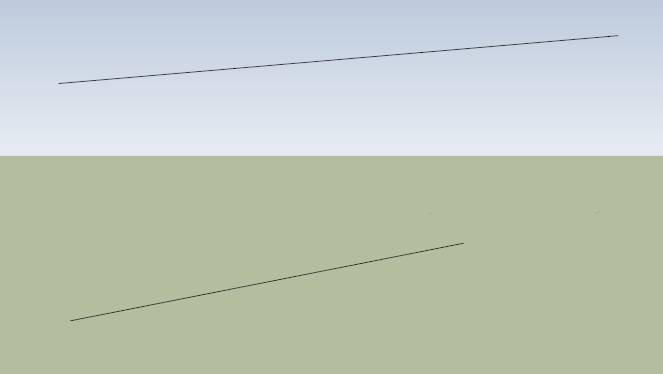# Intersecting lines without having to touch themAre there ways to intersect them without having to touch them? A plugin maybe?

Do you mean, you want to connect the closest points of the two selected edges?

Edges in the same context will break at the intersection, thereby create a mutual endpoint.
If you don’t want that to happen (visually) you’ll need to drop the upper edge in its own separate context.

• group the edge
• lower it along the blue axis to the lower edge
• close the group

You could create a guide using one of the edges and pull it vertically to the other edge to find their virtual intersection. Draw a vertical guide or edge to find the corresponding point on the other edge.

This too kludgy? Copy the upper line vertically down below the other, complete the plane by closing in the ends and the plane finds the intersection that lets you draw a vertical line.Works for both edges in horizontal planes, not a general solution with two arbitrary edges in space.

That is two arbitrary lines in space. Neither is horizontal. The plane is a parallelogram. (It’s hard to tell from one point of view)1 Like

(Watching TV now, will respond soon).
@RTCool, my earlier remark was just a copy of what I wrote in a response to @mihai.s where he showed two angles 90 degrees. Hobby mihai.s - #113 by mihai.s
Your method is correct if its about creating a vertical connecting edge.
I was thinking along the “line” of creating the shortes connecting edge between two arbitrary lines.

I hope OP will be back to explain what the real question is.

• intersection (my answer above which might be missing the point entirely)
• drap (in horizontal edges case, as shown by @mihai.s) and what you showed
• the shortest connecting edge (in all arbitrary situations)

If you select both edges and paste the following snippet into the console (and press enter) a guide point will appear at each edge at the closest point.

``````es = Sketchup.active_model.selection.grep(Sketchup::Edge)
return unless es.length == 2
ray0 =  [es.start.position, es.end.position - es.start.position]
ray1 =  [es.start.position, es.end.position - es.start.position]
pts = Geom.closest_points(ray0, ray1)
return unless pts
``````
1 Like

Yes, vertical is what I did. I don’t know how shortest distance could be figured graphically.

This is one way with basic tools, from start to … (not finished yet):

• copy upper edge to somewhere on the lower edge
• create pink triangle from these intersecting edges
• triple click the ‘Tape Measure’ tool on yellow face (the guide is the desired direction)
• move the guide to intersect the upper edge somewhere
• create the yellow face from intersecting upper edge and guide
• … (missing part, see if you can get there to the end)
• enlarge yellow face so the lower edge intersects it
• draw the connecting edge in yellow face parallel to the guide

This connecting edge crosses both the upper and lower edges at an angle of 90 degrees and has the shortest distance.

p.s. A few steps less (forget about the guide and the yellow face altogether)

• have the pink face created large enough
• use ‘Push/Pull’ on the pink face so one of its side face intersects the upper edge
(the body side edges run in the right direction)
• connect this intersection perpendicular down towards the lower edge

A lot a variation interpreting what the OP meant by “intersecting”!

Certainly.
(To me the shortest distance with basic tools was the challenge.)

(as an example)

1 Likelast picture is a top view
Sorry for the delay guys.
I made an easy example here: I want to find the “common point” of those lines without having to moving the line to intersect them so I can find the point where they cross each other

Yes, I was doing this but it takes time to do it. I wonder if there is an easier way to do it…I wish skp should automatically have this option when you just pass the cursor in the line, like on autocad for example…maybe in the next version. Wish they could hire me to give them creative ideas. =DI think there are plugins that will flatten the 3D model to one plane, so that might speed it up a little by compressing a copy to a flat plane where they would intersect and then draw up from there.

I wish we could drape a line at least…not only a mesh

I use TIG’s extrude edges by vector to quickly create a vertical plane from one line. Because it makes a group it is quick to erase and does not interfere with other geometry.
https://sketchucation.com/forums/viewtopic.php?f=323&t=37515

1 Like

Here 3 copies of 2 crossing edges: model file
Crossing edges 3D.skp (152.5 KB)

1. vertically projected “intersection” found/shown by sliding a section plane (Vertically)
1. shortest distance found/shown by sliding a section plane (Perpendicular to one edge)
1. exact shortest distance found by construction

p.s. In 1. and 2. you can slide the section plane to examine the shown distances